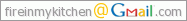## Saturday, November 18, 2006

### Converting Between Fahrenheit & Celsius (or Centigrade)

Converting Between Fahrenheit & Celsius (or Centigrade)
(Use for Tempature on the Oven)

Fahrenheit: Under standard conditions water boils at 212 degrees and freezes at 32 degrees
Celsius or Centigrade: Water boils at 100 deg. and freezes as 0 deg.

To convert Celsius into Fahrenheit: Multiply by 9, divide by 5 and add 32.

To convert Fahrenheit into Celsius: Subtract 32, multiply by 5 and divide by 9

Too Lazy? Try this link: Table Of Degrees Centigrade to Degrees Fahrenheit